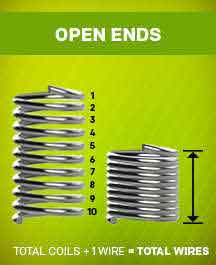<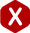# Solid Height

Solid height for compression springs is defined as the point at which all wires in your spring are touching and no more force can be exerted by the spring. Solid height plays are crucial role in compression spring design.

A compression spring may be capable of exerting great amounts of force but solid height can greatly decrease those numbers. The most classic example of a compression spring traveling to solid height is a pen. When the button on the end of the pen is pushed, the spring will travel to solid height, which will exert the amount of force needed to push the tip out of the pen. Similarly when the pen is pushed again the spring will release and return to its free state bringing the tip of the pen back into the body.#### Calculating Solid Height

To calculate solid height for your spring you will first need to know what type of ends you need on your spring. The most common and economical type of compression spring ends are closed and squared ends. If you spring needs to lay flat on a surface without the wobble effect that closed and square ends will cause you will need closed and ground ends. The ground ends will add an extra cost to your spring so make sure this is a necessary feature. If you need to thread the ends of your spring into something then you will need open ends on your compression spring. If your design required you to thread your spring onto a device or needs to be stable at the top and bottom you will need multiple closed ends, such as double closed.#### Calculating Solid Height - Closed And Square Ends:

The formula for calculating solid height on closed and square ends is:

Total Wires = Total Coils + 1
Solid Height = Total Wire * Wire Diameter

There are two ways to get the total amount of wires in a closed and square end compression spring. You can count them as in the picture to your right or you can take the total number of coils and add 1 if you just have the dimensions of the spring.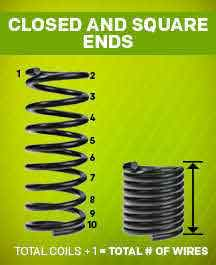#### Calculating Solid Height - Closed And Ground Ends:

The formula for calculating solid height on closed and ground ends is:

Total Wires = Total Coils
Solid Height = Total Wire * Wire Diameter

In a closed and ground compression spring, the solid height can be calculated using the formula above. In the case since the spring has been ground down by half a wire diameter on each side you do not have to add one wire diameter to the total coils to get the amount of wires in the spring. For closed and ground compression springs the total coils are equal to the total wires.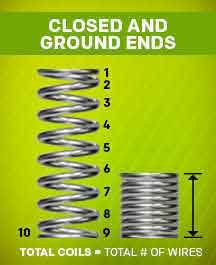#### Calculating Solid Height - Double Closed Ends:

The formula for calculating solid height on double closed ends is:

Total Wires = Total Coils + 1
Solid Height = Total Wire * Wire Diameter

The solid height for a compression spring with double closed ends works similarly to that of one with closed ends only. You can count the number of wires as in the picture to the right or you can take the total number of coils and add 1. This means that total coils + 1 Wire = total wires. Then take the total wire * (times) the wire diameter = solid height.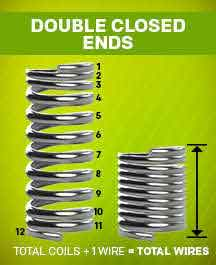#### Calculating Solid Height - Open Ends:

The formula for calculating solid height on open ends is:

Total Coils + 1 = Total Wires
Solid Height = Total Wire * Wire Diameter

The solid height for a compression spring with open ends works a little differently than the other three types of ends. Since the active coils on an open ended spring is the total coils on an open ended spring you need to add one wire diameter. This will get you the appropriate solid height.